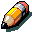This is a child page. You can use Parent in the quick nav bar at the top or the bottom of the page to navigate directly back to the parent of this page. Some child pages are more than one level deep, and in that case will require more than one Parent click to reach the outermost document level.

### § 16.69.5 - Advanced Concepts for Transition Control Files

F/x's transition generator can use a built-in mathematical expression evaluator.

In order to use this, the evaluator must be passed:

• An exclamation point (!) to begin the expression, adding this escape character is the only way an expression can be evaluated.
• Valid characters:
• Parentheses: ) and (
• Division and Multiplication: / and *
• Addition and Subtraction: + and -
• Power: ^
• Modulus: %
• Valid functions: (described below)
• The proper syntax when calling the time variable
• A properly formatted mathematical function.
• NO SPACES. A space designates that the argument is complete to the evaluator.Note:

The variable called by "ttime(0)" returns the "current time". In a timeline, this returns the percentage of the timeline completed or the time value for a still. (The current time value will be in the form of 0.0 to 1.0)

The expression evaluator will accept these functions:

FunctionDetails
abs(expression)Absolute Value
acos(expression)Arc-Cosine
asin(expression)Arc-Sine
atan(expression)Arc-Tangent
ceil(expression)Ceiling (nearest whole number > expression)
cframe(0)Returns current frame as number 1 to tframes()
cos(expression)Cosine
cosh(expression) Hyperbolic Cosine
exp(expression)Exponential
floor(expression)Floor (nearest whole number < expression)
hyp(expression,expression)Hypotenuse of X, Y
linv(expression)Takes frame number as parameter, returns linear value 0 to (tframes(0)) as 0-1
log(expression)Natural Log
log10(expression)Log, base 10
loopv(expression)Takes frame number as parameter, returns linear value 0 to (tframes(0)+1) as 0-1
sin(expression)Sine
sinh(expression)Hyperbolic Sine
sqrt(expression)Square Root
tan(expression)Tangent
tanh(expression)Hyperbolic Tangent
tframes(0)Returns total number of frames in the sequence
ttime(0)Returns the current value for time in the range 0 to one.

An example using the expression evaluator